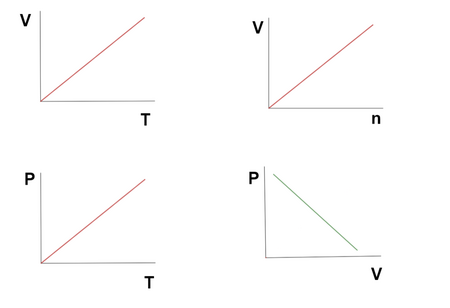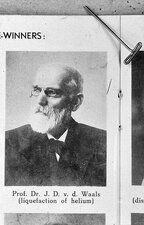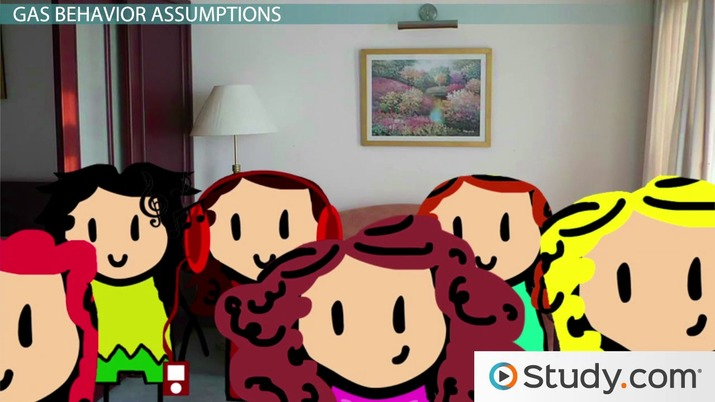# Real Gases vs. Ideal Gas Laws

• Author

A freelance tutor equipped with a bachelor's of science in chemical engineering. Graduated from the American University of the Middle East with a GPA of 3.87, performed a number of scientific primary and secondary research. Tutored university level students in various courses in chemical engineering, math, and art. Has experience tutoring middle school and high school level students in science courses.

• Instructor
Elizabeth Friedl

Elizabeth, a Licensed Massage Therapist, has a Master's in Zoology from North Carolina State, one in GIS from Florida State University, and a Bachelor's in Biology from Eastern Michigan University. She has taught college level Physical Science and Biology.

Understand the ideal gas law while examining ideal gas behavior assumptions and conditions, the Van Der Waals equation, and the deviations from the ideal gas laws. Updated: 12/02/2021

Show

## Ideal Gas Behavior

Ideal gas behaviors show that the molecules of ideal gases do not lose energy upon traveling and colliding with other molecules. An ideal gas is a theoretical gas that obeys the ideal gas law and whose individual molecules occupy negligible space. The molecules of ideal gases collide elastically and exhibit no resistance to flow. The energy of these molecules is conserved because of the elastic and frictionless nature of their movements. Ideal gases have neither intermolecular forces nor attraction between their molecules; the distance between each molecule is by magnitudes larger than the size of the single molecule. The ideal gas law is a law that governs the behavior of ideal gases; it shows the relationship between the following macroscopic properties: the gas's volume, temperature, and pressure. The ideal gas law formula is:

{eq}PV = nRT {/eq}

Where:

• P is the pressure.
• V is the volume.
• n is the number of moles of gas.
• R is the universal gas constant; its value is dependent on its unit.
• T is the temperature. The temperature must always be absolute (in Kelvins).

The following example shows how this formula can be applied. At what temperature would 1.3 moles of oxygen have a pressure of 1.4 atm in a 30L vessel?

{eq}PV = nRT \rightarrow 1.4 atm * 30 L = 1.3 mol * 0.0826 (L \: atm/K \: mol) * T {/eq}

Solving for {eq}T = 391.13 K {/eq}.

What does the ideal gas law describe? It describes the behavior of hypothetical gases. Why is studying the behavior of fictional gases important? The importance stems from the fact that studying the behavior of ideal gases is the key to understanding how real gases behave. The ideal gas law shows how the change in the pressure of a gas impacts the other parameters. The formula shows the following:

• At a fixed amount of gas (moles) and at a constant volume, the pressure and the temperature are directly proportional to one another.
• At a fixed amount of gas (moles) and at a constant temperature, the volume and the pressure are inversely related to one another.
• At a fixed amount of gas (moles) and at constant pressure, the temperature and the volume are directly proportional to one another.
• At a constant temperature and pressure, the amount of gas is directly proportional to its volume.

Figure 1 summarizes the relationship between each variable in the ideal gas equation. These relationships were discovered by different scientists. Their combination resulted in the formation of the ideal gas law.The ideal gas law is only applicable under certain 'ideal' conditions which are discussed in the following subsection.

### Ideal Gas Assumptions

The ideal gas equation is applicable under very specific conditions; the following are the ideal gas law conditions:

• When the intermolecular forces between the molecules are insignificant.
• When the volume of the individual gas molecule is negligible.
• The collisions between the gas molecules and between the molecules and the walls of the vessel are completely elastic.
• The motion of the gas molecules is random and continuous. The gas molecules travel in a straight-line motion.
• When the gas density is low; inter-particle attractions are negligible.

The ideal gas assumptions were first recognized by the Dutch scientist Johannes Van der Waals. The two main assumptions that segregate ideal gases from real gases are:

1. The volume of individual molecules of an ideal gas is negligible; they are very small to the point they (individually) occupy no space.
2. The intermolecular attraction between the gas particles is negligible.

Consider a gas sample that is trapped in a compressed tank. As the volume inside the tank decreases, the intermolecular forces between the gas molecules become more significant. This is a direct consequence of compressing the gas molecules and making them close to one another. In this instance the ideal gas law fails; the intermolecular attraction is too significant to neglect. The ideal gas law becomes invalid when:

• The distance between each gas molecule is small; the intermolecular forces become significant when the molecules are close to one another.

Therefore, it can be deduced that the deviations from the ideal gas law occur at high pressures and at low temperatures; when the pressure is high the gas molecules are compressed and the intermolecular forces become too significant to neglect. The reason why the law fails at low temperatures is that the molecules' kinetic energy is severely compromised, which means the molecules travel at a slower pace. The decrease in the velocities of the molecules results in the gas particles being close to one another, which again increases the effect of the intermolecular attractions. Gases with high densities do not behave ideally because of the significant intermolecular forces between their particles.An error occurred trying to load this video.

Try refreshing the page, or contact customer support.

Coming up next: Real Gases: Using the Van der Waals Equation

### You're on a roll. Keep up the good work!

Replay
Your next lesson will play in 10 seconds
• 0:07 The Ideal Gas Law
• 2:04 Gas Behavior Assumptions
• 4:17 The van der Waals Equation
• 6:18 Lesson Summary
Save Save

Want to watch this again later?

Timeline
Autoplay
Autoplay
Speed Speed

To unlock this lesson you must be a Study.com Member.

#### What are the main characteristics of an ideal gas?

The main characteristics of ideal gases are the following:

• The intermolecular attraction between the gas particles is negligible because the distance between the gas molecules is very large.
• The individual molecules occupy no volume in space.
• The particle-particle collisions and the particle-container collisions are elastic.
• Gas molecules are at constant random motion. They travel in a straight line.

#### Why are real gases not ideal?

Real gases deviate from the ideal gas behavior for the following reasons:

• The volume of the individual gas molecules cannot be neglected. The individual gas molecules occupy an actual volume in space.
• The inter-particles' attractive and repulsive forces are significant and cannot be overlooked.

Gases deviate from the ideal behavior at high pressures, low temperatures, and high densities.

### Register to view this lesson

Are you a student or a teacher?